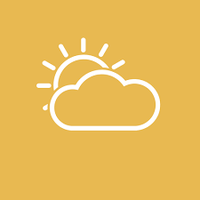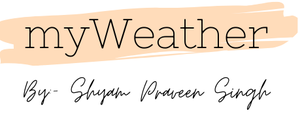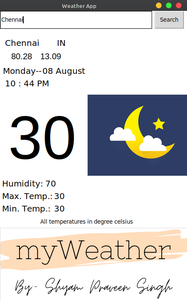# Create a GUI for Weather Forecast using openweathermap API in Python

The idea of this article is to provide a simple GUI application to users to get the current temperature of any city they wish to see. The system also provides a simple user interface for simplification of application. It also provides an amazing UX for its users. The features of this application will be that this will be a real-time weather forecast app that returns the current temperature, maximum and minimum temperature, humidity, latitude, and longitude coordinates of the searched city, current date, and time. It can also change its theme according to the time of day.

### Openweathermap API

openweathermap is a service that provides weather data, including current weather data, forecasts, and historical data to the developers of web services and mobile applications.

### Modules Needed

• Tkinter: It is the fastest and easiest way to create GUI applications. This is included in the Python standard modules so there is no need to install it externally.
• PIL: PIL is the Python Imaging Library which provides the python interpreter with image editing capabilities.
• json: This module is used to handle JSON files and comes built in with Python. So there is no need to install it externally
• requests: It is used for making HTTP requests to a specified URL. This module does not comes built in with Python. To install it type the below command in the terminal.
`pip install requests`

Images Used in the below application:moon.pngsun.pnglogo.png

Below is the implementation.

## Python3

 `# python3 -- Weather Application using API ` ` `  `# importing the libraries ` `from` `tkinter ``import` `*` `import` `requests ` `import` `json ` `import` `datetime ` `from` `PIL ``import` `ImageTk, Image ` ` `  ` `  `# necessary details ` `root ``=` `Tk() ` `root.title(``"Weather App"``) ` `root.geometry(``"450x700"``) ` `root[``'background'``] ``=` `"white"` ` `  `# Image ` `new ``=` `ImageTk.PhotoImage(Image.``open``(``'logo.png'``)) ` `panel ``=` `Label(root, image``=``new) ` `panel.place(x``=``0``, y``=``520``) ` ` `  ` `  `# Dates ` `dt ``=` `datetime.datetime.now() ` `date ``=` `Label(root, text``=``dt.strftime(``'%A--'``), bg``=``'white'``, font``=``(``"bold"``, ``15``)) ` `date.place(x``=``5``, y``=``130``) ` `month ``=` `Label(root, text``=``dt.strftime(``'%m %B'``), bg``=``'white'``, font``=``(``"bold"``, ``15``)) ` `month.place(x``=``100``, y``=``130``) ` ` `  `# Time ` `hour ``=` `Label(root, text``=``dt.strftime(``'%I : %M %p'``), ` `             ``bg``=``'white'``, font``=``(``"bold"``, ``15``)) ` `hour.place(x``=``10``, y``=``160``) ` ` `  `# Theme for the respective time the application is used ` `if` `int``((dt.strftime(``'%I'``))) >``=` `8` `& ``int``((dt.strftime(``'%I'``))) <``=` `5``: ` `    ``img ``=` `ImageTk.PhotoImage(Image.``open``(``'moon.png'``)) ` `    ``panel ``=` `Label(root, image``=``img) ` `    ``panel.place(x``=``210``, y``=``200``) ` `else``: ` `    ``img ``=` `ImageTk.PhotoImage(Image.``open``(``'sun.png'``)) ` `    ``panel ``=` `Label(root, image``=``img) ` `    ``panel.place(x``=``210``, y``=``200``) ` ` `  ` `  `# City Search ` `city_name ``=` `StringVar() ` `city_entry ``=` `Entry(root, textvariable``=``city_name, width``=``45``) ` `city_entry.grid(row``=``1``, column``=``0``, ipady``=``10``, stick``=``W``+``E``+``N``+``S) ` ` `  ` `  `def` `city_name(): ` ` `  `    ``# API Call ` `    ``api_request ``=` `requests.get(``"https://api.openweathermap.org/data/2.5/weather?q="` `                               ``+` `city_entry.get() ``+` `"&units=metric&appid="``+``api_key) ` ` `  `    ``api ``=` `json.loads(api_request.content) ` ` `  `    ``# Temperatures ` `    ``y ``=` `api[``'main'``] ` `    ``current_temprature ``=` `y[``'temp'``] ` `    ``humidity ``=` `y[``'humidity'``] ` `    ``tempmin ``=` `y[``'temp_min'``] ` `    ``tempmax ``=` `y[``'temp_max'``] ` ` `  `    ``# Coordinates ` `    ``x ``=` `api[``'coord'``] ` `    ``longtitude ``=` `x[``'lon'``] ` `    ``latitude ``=` `x[``'lat'``] ` ` `  `    ``# Country ` `    ``z ``=` `api[``'sys'``] ` `    ``country ``=` `z[``'country'``] ` `    ``citi ``=` `api[``'name'``] ` ` `  `    ``# Adding the received info into the screen ` `    ``lable_temp.configure(text``=``current_temprature) ` `    ``lable_humidity.configure(text``=``humidity) ` `    ``max_temp.configure(text``=``tempmax) ` `    ``min_temp.configure(text``=``tempmin) ` `    ``lable_lon.configure(text``=``longtitude) ` `    ``lable_lat.configure(text``=``latitude) ` `    ``lable_country.configure(text``=``country) ` `    ``lable_citi.configure(text``=``citi) ` ` `  ` `  `# Search Bar and Button ` `city_nameButton ``=` `Button(root, text``=``"Search"``, command``=``city_name) ` `city_nameButton.grid(row``=``1``, column``=``1``, padx``=``5``, stick``=``W``+``E``+``N``+``S) ` ` `  ` `  `# Country  Names and Coordinates ` `lable_citi ``=` `Label(root, text``=``"..."``, width``=``0``,  ` `                   ``bg``=``'white'``, font``=``(``"bold"``, ``15``)) ` `lable_citi.place(x``=``10``, y``=``63``) ` ` `  `lable_country ``=` `Label(root, text``=``"..."``, width``=``0``,  ` `                      ``bg``=``'white'``, font``=``(``"bold"``, ``15``)) ` `lable_country.place(x``=``135``, y``=``63``) ` ` `  `lable_lon ``=` `Label(root, text``=``"..."``, width``=``0``, ` `                  ``bg``=``'white'``, font``=``(``"Helvetica"``, ``15``)) ` `lable_lon.place(x``=``25``, y``=``95``) ` `lable_lat ``=` `Label(root, text``=``"..."``, width``=``0``, ` `                  ``bg``=``'white'``, font``=``(``"Helvetica"``, ``15``)) ` `lable_lat.place(x``=``95``, y``=``95``) ` ` `  `# Current Temperature ` ` `  `lable_temp ``=` `Label(root, text``=``"..."``, width``=``0``, bg``=``'white'``, ` `                   ``font``=``(``"Helvetica"``, ``110``), fg``=``'black'``) ` `lable_temp.place(x``=``18``, y``=``220``) ` ` `  `# Other temperature details ` ` `  `humi ``=` `Label(root, text``=``"Humidity: "``, width``=``0``,  ` `             ``bg``=``'white'``, font``=``(``"bold"``, ``15``)) ` `humi.place(x``=``3``, y``=``400``) ` ` `  `lable_humidity ``=` `Label(root, text``=``"..."``, width``=``0``, ` `                       ``bg``=``'white'``, font``=``(``"bold"``, ``15``)) ` `lable_humidity.place(x``=``107``, y``=``400``) ` ` `  ` `  `maxi ``=` `Label(root, text``=``"Max. Temp.: "``, width``=``0``,  ` `             ``bg``=``'white'``, font``=``(``"bold"``, ``15``)) ` `maxi.place(x``=``3``, y``=``430``) ` ` `  `max_temp ``=` `Label(root, text``=``"..."``, width``=``0``,  ` `                 ``bg``=``'white'``, font``=``(``"bold"``, ``15``)) ` `max_temp.place(x``=``128``, y``=``430``) ` ` `  ` `  `mini ``=` `Label(root, text``=``"Min. Temp.: "``, width``=``0``,  ` `             ``bg``=``'white'``, font``=``(``"bold"``, ``15``)) ` `mini.place(x``=``3``, y``=``460``) ` ` `  `min_temp ``=` `Label(root, text``=``"..."``, width``=``0``,  ` `                 ``bg``=``'white'``, font``=``(``"bold"``, ``15``)) ` `min_temp.place(x``=``128``, y``=``460``) ` ` `  ` `  `# Note ` `note ``=` `Label(root, text``=``"All temperatures in degree celsius"``, ` `             ``bg``=``'white'``, font``=``(``"italic"``, ``10``)) ` `note.place(x``=``95``, y``=``495``) ` ` `  ` `  `root.mainloop() `

Output:Attention geek! Strengthen your foundations with the Python Programming Foundation Course and learn the basics.

To begin with, your interview preparations Enhance your Data Structures concepts with the Python DS Course.

My Personal Notes arrow_drop_upCheck out this Author's contributed articles.

If you like GeeksforGeeks and would like to contribute, you can also write an article using contribute.geeksforgeeks.org or mail your article to contribute@geeksforgeeks.org. See your article appearing on the GeeksforGeeks main page and help other Geeks.

Please Improve this article if you find anything incorrect by clicking on the "Improve Article" button below.

Article Tags :

1

Please write to us at contribute@geeksforgeeks.org to report any issue with the above content.